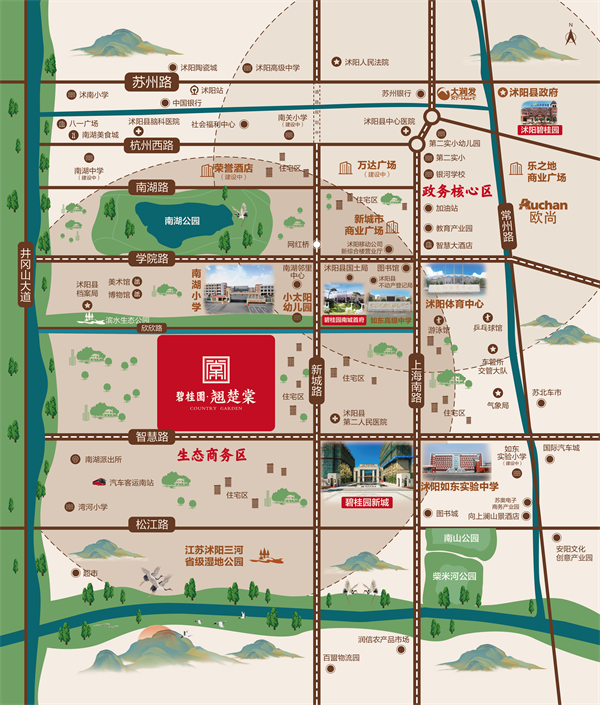## 网站地图## 碧桂园·翘楚棠（宿迁）

### 宿迁

#### 地址

• 资阳
• 本溪
• 北流
• 万载
• 慈溪
• 长春
• 通化
• 西昌
• 丰城
• 阳春
• 鹤山
• 江油
• 辛集
• 辽源
• 衡水
• 黔西南州
• 黔东南州
• 石家庄
• 唐山
• 秦皇岛
• 邯郸
• 邢台
• 保定
• 张家口
• 承德
• 沧州
• 廊坊
• 黄骅
• 迁安
• 怀来
• 洛阳
• 驻马店
• 郑州
• 开封
• 平顶山
• 安阳
• 鹤壁
• 新乡
• 焦作
• 濮阳
• 许昌
• 漯河
• 三门峡
• 南阳
• 商丘
• 信阳
• 周口
• 武汉
• 黄石
• 十堰
• 宜昌
• 襄阳
• 荆州
• 荆门
• 鄂州
• 仙桃
• 潜江
• 黄冈
• 随州
• 咸宁
• 恩施
• 怀化
• 长沙
• 株洲
• 湘潭
• 湘西州
• 张家界
• 益阳
• 郴州
• 衡阳
• 岳阳
• 常德
• 娄底
• 徐州
• 南京
• 无锡
• 镇江
• 泰州
• 淮安
• 舟山
• 黔西南布依族苗族自治州
• 临沂
• 凉山
• 普洱
• 红河
• 锦州
• 葫芦岛
• 东方
• 咸阳
• 丽水
• 定安县
• 吕梁
• 来宾
• 河池
• 宜宾
• 重庆市
• 雅安
• 潮州
• 大理
• 巴中
• 桂林
• 贵港
• 百色
• 柳州
• 贺州
• 儋州
• 福清
• 威海
• 烟台
• 乐山
• 金华
• 永泰
• 潍坊
• 滨州
• 德州
• 临汾
• 日照
• 眉山
• 武威
• 乌鲁木齐
• 保山
• 临沧
• 防城港
• 达州
• 遂宁
• 绵阳
• 淮南
• 宝鸡
• 枣庄
• 桂平
• 景德镇
• 临夏
• 聊城
• 崇左
• 马鞍山
• 安顺
• 北海
• 菏泽
• 铜陵
• 黔南州
• 蚌埠
• 嘉兴
• 淮北
• 都匀
• 南宁
• 兴义
• 南平
• 松溪
• 吉安
• 大同
• 陆丰
• 厦门
• 铜仁
• 银川
• 昭通
• 包头
• 遵化
• 漳州
• 深圳
• 霸州
• 香港
• 合肥
• 抚州
• 琼海
• 定西
• 南昌
• 宿州
• 文昌
• 临高县
• 陵水
• 海口
• 博鳌
• 三亚
• 绥化
• 哈尔滨
• 杭州
• 绍兴
• 宁波
• 温州
• 衢州
• 湖州
• 台州
• 天津
• 沈阳
• 大连
• 通辽
• 兴安盟
• 呼伦贝尔
• 运城
• 晋中
• 太原
• 南通
• 宿迁
• 扬州
• 盐城
• 连云港
• 苏州
• 常州
• 安庆
• 黄山
• 巢湖
• 芜湖
• 池州
• 宣城
• 六安
• 古田
• 无为
• 桐城
• 亳州
• 南陵
• 庐江
• 阜阳
• 滁州
• 孝感
• 天门
• 邵阳
• 永州
• 平果
• 玉林
• 梧州
• 钦州
• 梅州
• 河源
• 云浮
• 东莞
• 汕头
• 中山
• 清远
• 惠州
• 韶关
• 肇庆
• 阳江
• 汕尾
• 茂名
• 江门
• 佛山
• 广州
• 雷州
• 英德
• 湛江
• 揭阳
• 珠海
• 信宜
• 济南
• 淄博
• 青岛
• 泰安
• 济宁
• 马来西亚雪兰莪州
• 马来西亚柔佛州
• 永春
• 南安
• 三明
• 宁德
• 武夷山
• 福鼎
• 莆田
• 建瓯
• 漳浦
• 福安
• 福州
• 泉州
• 龙岩
• 兰州
• 张掖
• 天水
• 白银
• 贵阳
• 仁怀
• 毕节
• 凯里
• 六盘水
• 遵义
• 广元
• 德阳
• 南充
• 泸州
• 攀枝花
• 广安
• 成都
• 宜春
• 萍乡
• 九江
• 赣州
• 上饶
• 曲靖
• 楚雄
• 昆明
• 白城
• 北京
• 澳洲莱德
• 西安
• 渭南
• 上海
• 西宁

傲居南城 缔造人居范本

碧桂园·翘楚棠位于沭阳南部新城板块，政府规划“南进、东拓、西控、北优”的发展战略，将南城区设计“八横三纵”主框架，未来城市主要发展方向，也是沭阳重点打造城南生态新城板块，集交通环境，人文教育，自然环境于一身。碧桂园·翘楚棠萃集27年筑家精髓，延续城市文脉，筑造沭阳的品质人文住区。

阳光房企，责任地产

碧桂园集团成立于1992年，深耕地产业27载，开发逾1200个城镇，超400万业主一致选择，是国内实力地产企业之一。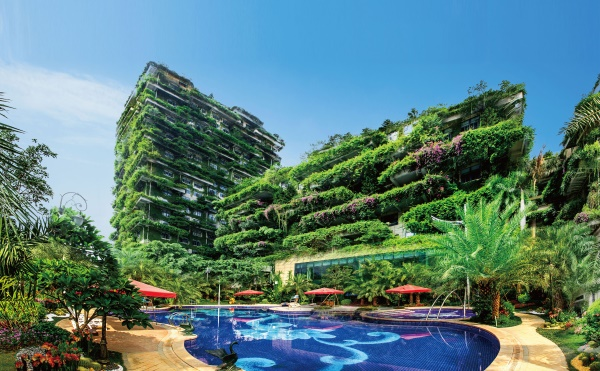公园环伺，天然绿肺

项目南侧为滨水生态公园，风景优美，奢享自然生态风光;3公里内享三河湿地公园(在建中)、南山公园、南湖公园、柴米河湾生态公园等生态矩阵萦绕，多园围合之势，筑造醉美富氧公园大境;在璀璨四季里，与家人沉醉于美景之中。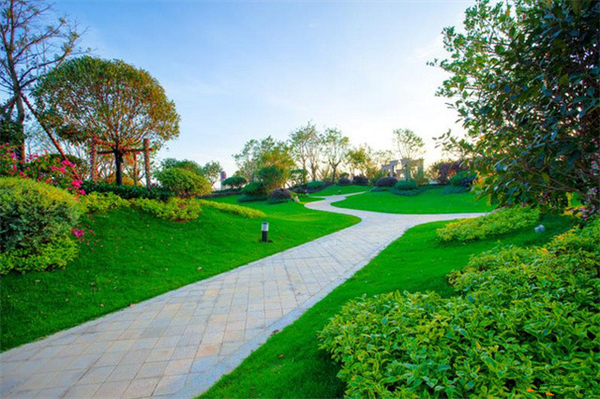名校聚集 人文圣地

项目一河之隔南湖小学、小太阳幼儿园，周边3公里范围内如东高级中学、如东实验初中等优质学校集聚，浓厚人文熏陶，助力孩子锦绣前程。

景观怡心 典藏四季

秉承传统技艺，运用现代元素，于外博取自然之妙，于内一览草木之趣，浓墨重彩地再现新亚洲园林风雅大境，四季景观皆藏于园中，生活于此共赏春花秋月，演绎城市诗意生活新篇章。

贴心服务，2018物业三强

物业服务乘承创始人“急业主所急，想业主所想”的寄语，整合众多高端楼盘优质服务理念，打造雅生活服务体系。专业高效的管理经验，24小时管家式服务，带给业主更细致、更专业的服务体验。亲情化和充满人文关怀，让您真正体验到碧桂园的用心、细心和贴心。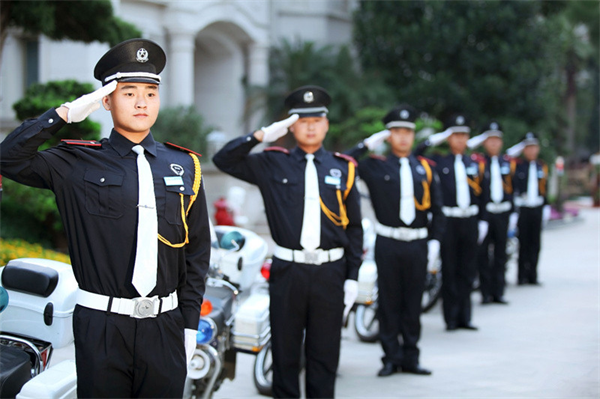新亚洲美宅 尽显人居美学

采用新亚洲建筑风格，融东方典雅意境与现代建筑理念于一体，凝萃人性化的设计考量，以现代人的审美需求及科学的布局、阔绰的尺度、舒适的人居体验，为沭阳人文住宅，盛启崭新篇章。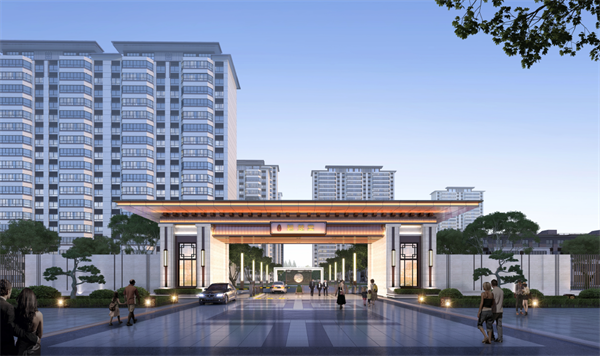项目区位示意图：